## Introduction

This package was first designed to set break points to truncate plot as I need to shrink outlier long branch of a phylogenetic tree.

Axis break or a so call gap plot is useful for data are not normal and contain outliers. Sometimes we can transform the data (e.g. using log if the data was log normal distributed) to solve this problem. But this is not always granted. The data may just simply contains outliers and these outliers are meaningful. A simple gap plot can solve this issue well to present the data in details with both normal and extreme data.

This package provides several scale functions to break down a ‘gg’ plot into pieces and align them together with (gap plot) or without (wrap plot or cut plot) ignoring subplots. Our methods are fully compatible with ggplot2, so that after breaking axis, users can still use + operator to add geometric layers.

## Gap plot

For creating gap plot, we only provide scale_x_break and scale_y_break functions. Currently applying both function to set break points for both axes are not allowed. However, multiple break points on a single axis are supported.

### Feature 1: Compatible with ggplot2.

After breaking the plot, we can still add geometric layers and setting themes.

library(ggplot2)
library(ggbreak)
library(patchwork)

set.seed(2019-01-19)
d <- data.frame(x = 1:20,
y = c(rnorm(5) + 4, rnorm(5) + 20, rnorm(5) + 5, rnorm(5) + 22)
)

p1 <- ggplot(d, aes(y, x)) + geom_col(orientation="y")
d2 <- data.frame(x = c(2, 18), y = c(7, 26), label = c("hello", "world"))
p2 <- p1 + scale_x_break(c(7, 17)) +
geom_text(aes(y, x, label=label), data=d2, hjust=1, colour = 'firebrick')  +
xlab(NULL) + ylab(NULL) + theme_minimal()

p1 + p2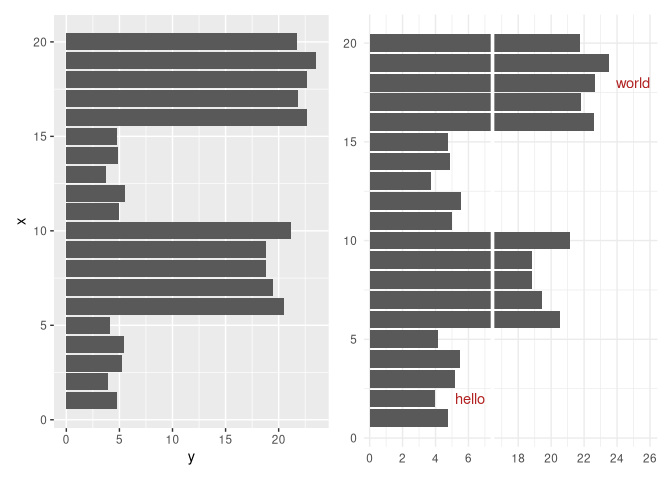### Feature 2: Multiple break points supported

p2 + scale_x_break(c(18, 21))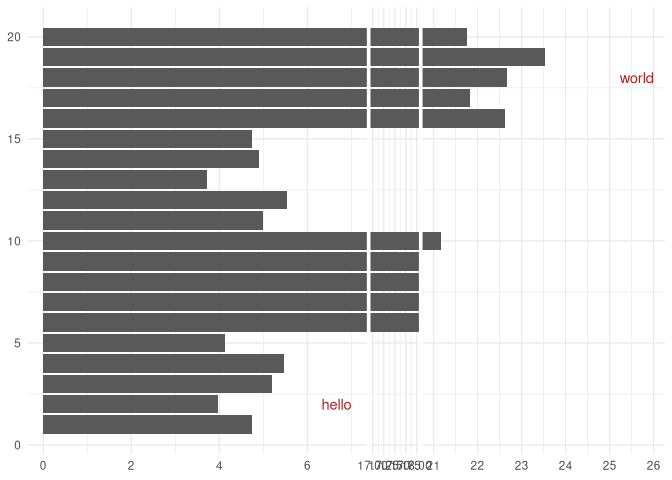### Feature 3: Zoom in or zoom out of subplots

p1 + scale_x_break(c(7, 17), scales = 1.5) + scale_x_break(c(18, 21), scales=2)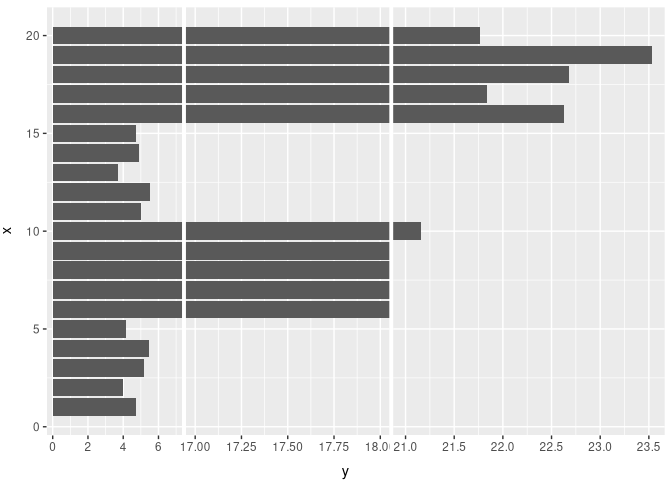### Feature 4: Support reverse scale

g <- ggplot(d, aes(x, y)) + geom_col()
g2 <- g + scale_y_break(c(7, 17), scales = 1.5) +
scale_y_break(c(18, 21), scale=2) + scale_y_reverse()
g + g2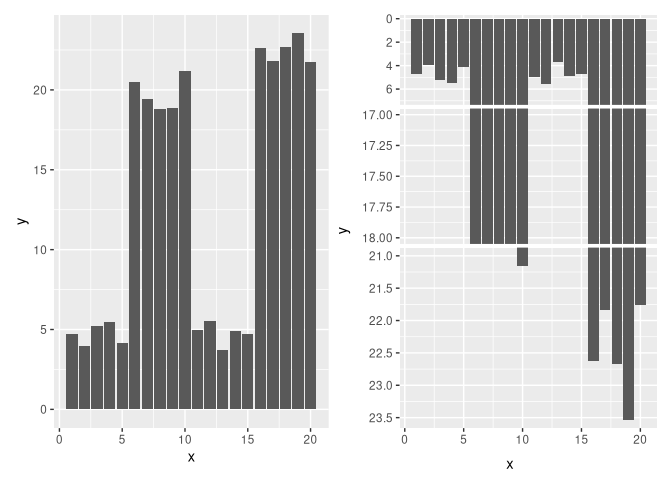### Feature 5: Compatible with scale transform functions

Users can apply scale transform functions, such as scale_x_log10 and scale_x_sqrt, to axis break plot.

p2 <- p1 + scale_x_break(c(7, 17))
p3 <- p1 + scale_x_break(c(7, 17)) + scale_x_log10()
p2 + p3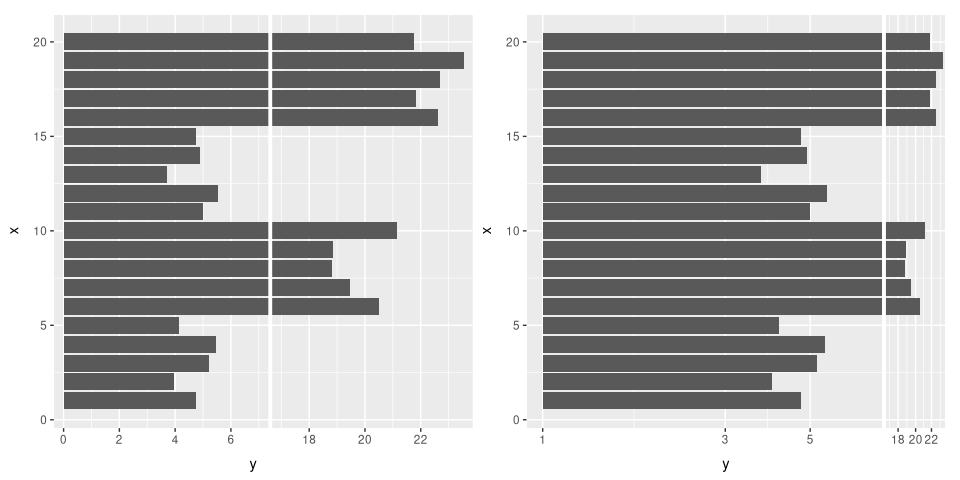### Feature 6: Compatible with coord_flip

g + coord_flip() + scale_y_break(c(7, 18))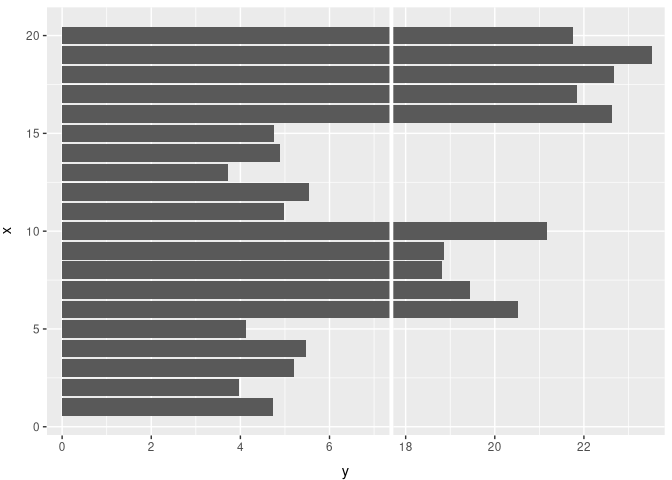### Feature 7: Compatible with facet_grid and facet_wrap

set.seed(2019-01-19)
d <- data.frame(
x = 1:20,
y = c(rnorm(5) + 4, rnorm(5) + 20, rnorm(5) + 5, rnorm(5) + 22),
group = c(rep("A", 10), rep("B", 10)),
face=c(rep("C", 5), rep("D", 5), rep("E", 5), rep("F", 5))
)

p <- ggplot(d, aes(x=x, y=y)) +
geom_col(orientation="x") +
scale_y_reverse() +
facet_wrap(group~.,
scales="free_y",
strip.position="right",
nrow=2
) +
coord_flip()
pg <- p +
scale_y_break(c(7, 17), scales="free") +
scale_y_break(c(19, 21), scales="free")
print(pg)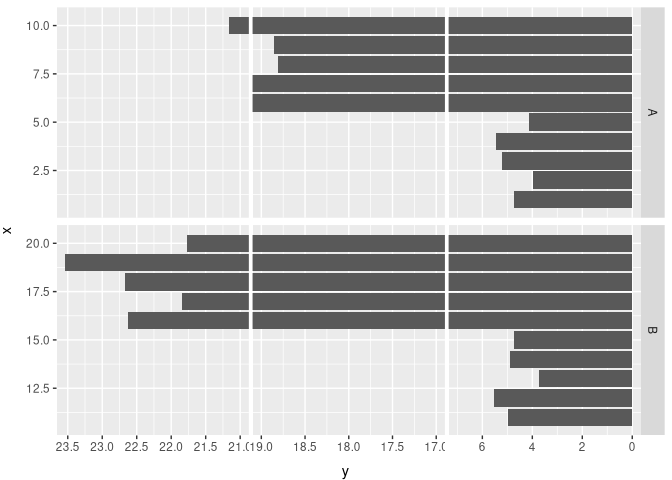### Feature 8: Compatible with legend

pg <- pg + aes(fill=group)
print(pg)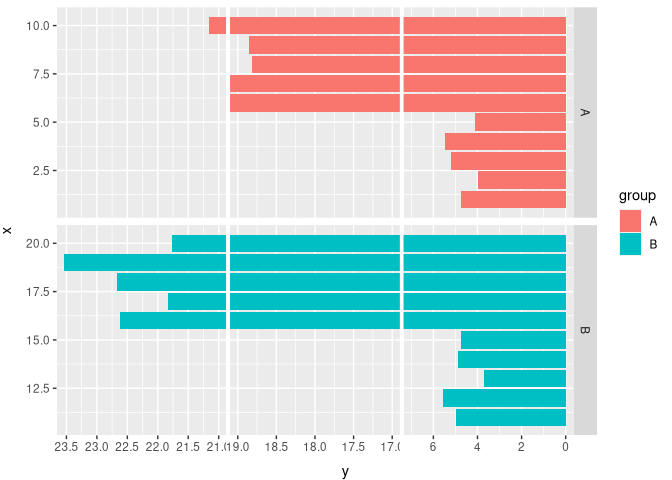### Feature 9: Supports all plot labels

pg + labs(title="test title", subtitle="test subtitle", tag="A tag", caption="A caption") +
theme_bw() +
theme(
legend.position = c(0.8, 0.8),
strip.placement = "outside",
axis.title.x=element_text(size=10),
plot.title = element_text(size = 22),
plot.subtitle = element_text(size = 16),
plot.tag = element_text(size = 10),
plot.title.position = "plot",
plot.tag.position = "topright",
plot.caption = element_text(face="bold.italic"),

)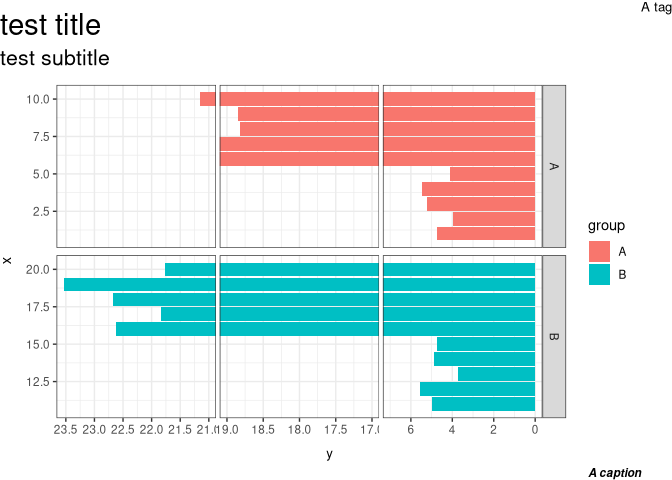### Feature 10: Allows setting tick labels for subplots

require(ggplot2)
library(ggbreak)
set.seed(2019-01-19)
d <- data.frame(
x = 1:20,
y =  c(rnorm(5) + 4, rnorm(5) + 20, rnorm(5) + 5, rnorm(5) + 22),
group = c(rep("A", 10), rep("B", 10))
)

p <- ggplot(d, aes(x=x, y=y)) +
scale_y_reverse() +
scale_x_reverse() +
geom_col(aes(fill=group)) +
scale_fill_manual(values=c("#00AED7", "#009E73")) +
facet_wrap(
group~.,
scales="free_y",
strip.position="right",
nrow=2
) +
coord_flip()

p +
scale_y_break(c(7, 10), scales=0.5, ticklabels=c(10, 11.5, 13)) +
scale_y_break(c(13, 17), scales=0.5, ticklabels=c(17, 18, 19)) +
scale_y_break(c(19,21), scales=1, ticklabels=c(21, 22, 23))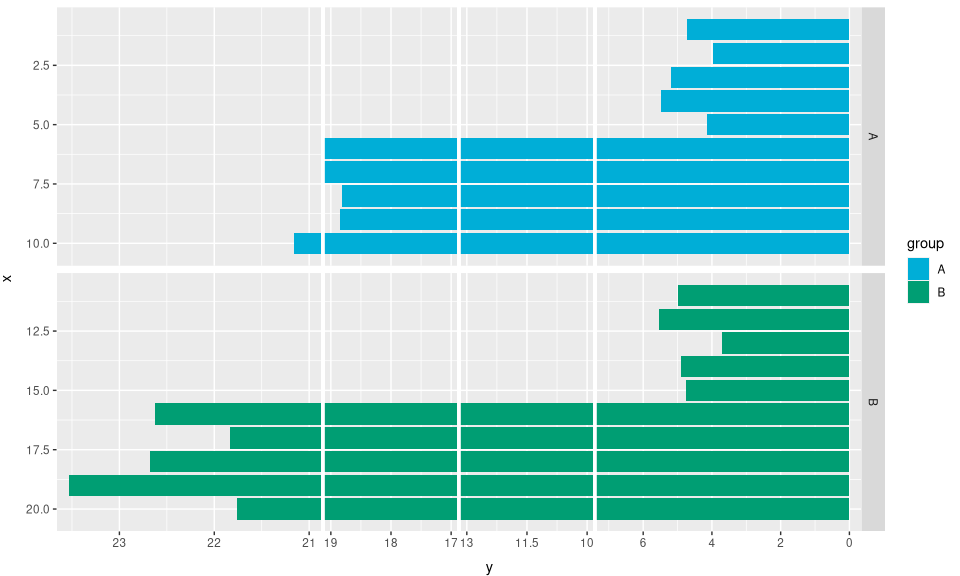### Feature 11: Compatible with patchwork

library(patchwork)

set.seed(2019-01-19)
d <- data.frame(
x = 1:20,
y = c(rnorm(5) + 4, rnorm(5) + 20, rnorm(5) + 5, rnorm(5) + 22)
)

p <- ggplot(d, aes(x, y)) + geom_col()
x <- p+scale_y_break(c(7, 17 ))

x + p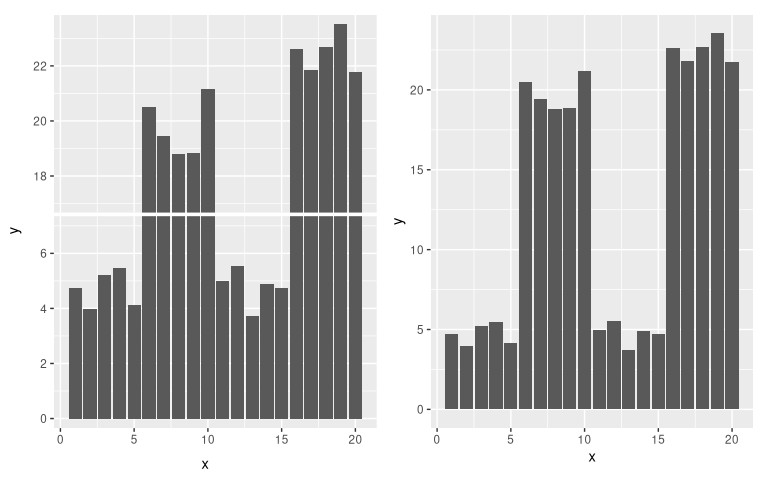## Wrap plot

The scale_wrap() function wraps a ‘gg’ plot over multiple rows to make plots with long x axes easier to read.

p <- ggplot(economics, aes(x=date, y = unemploy, colour = uempmed)) +
geom_line()

p + scale_wrap(n=4)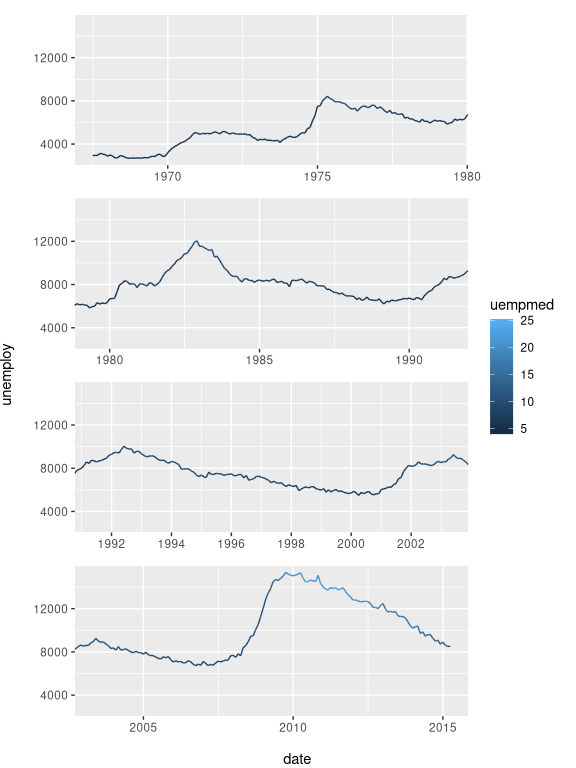## Cut plot

The scale_x_cut or scale_y_cut cuts a ‘gg’ plot to several slices with the ability to specify which subplots to zoom in or zoom out.

library(ggplot2)
library(ggbreak)
set.seed(2019-01-19)
d <- data.frame(
x = 1:20,
y = c(rnorm(5) + 4, rnorm(5) + 20, rnorm(5) + 5, rnorm(5) + 22)
)
p <- ggplot(d, aes(x, y)) + geom_col()
p + scale_y_cut(breaks=c(7, 18), which=c(1, 3), scales=c(3, 0.5))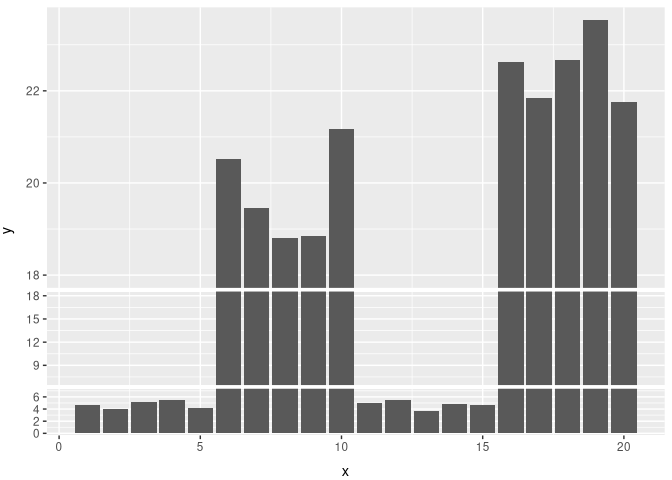## Note

The features we introduced for scale_x_break and scale_y_break also work for scale_wrap, scale_x_cut and scale_y_cut.